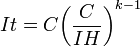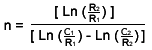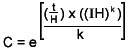BatteryStuff.com
Knowledge Base : Tools : Math Behind the Magic

# Math Behind the Magic

Here are the formulas our calculators take into consideration while computing.  What?  You thought we would show our work step by step?  You’re welcome to do it longhand if you like, but personally, I like my calculators better.

### Peukert’s Law (As we use it in Finding Load Specific Run Time)H is the rated discharge time, in (hours).

C is the rated capacity at that discharge rate, in (Ampere-hours).

I is the actual discharge current, in (Amps).

k is the Peukert constant, (dimensionless).

t is the actual time to discharge the battery, in (hours).

To find the Peukert constant, we must have two different ratings from the manufacturer.  Most manufacturers will give a 20 hour rate AH rating as the standard rating, and also a 10 hour rate and possibly a 100 hour rate.  With two of these, you can figure out Peukert’s number using the simple (J maniacal laughter) formula below.n = Peukert’s constant

C1 = Capacity at 1st rate

C2 = Capacity at 2nd rate

R1 = hour rate at 1st C rating

R2 = hour rate at 2nd C rating

Or, you can use our calculator and avoid a headache. Find it here.

Here is gets complicated.  In this formula we are trying to solve for C, the Rated capacity at a 20hr rate....Yeah, just use the Calculator

### Converting AC to DC

This one is simple, it’s Ohm's Law.

## W = V x A

Wattage = Voltage x Amperage

or as we use it:

Amperage = Wattage / Voltage

Email address should be formatted user@domain.com
We respect your rights to privacy and will not share your email information with anyone ever.
5 people commented, Prfcdoc, Steve, Cr0w, Gbadamosi Bamidele, and 1 other

• Don’t know if the author is still around. It’s been several years since this article was posted, but I love it. I’m an old school nerd with a sense of humor, clawing my way into the 21st Century. Was in the slide rule club in high school.

Reply  •  Ratted article 5  •  May 1, 2016 at 5:37 am
• Love it. Now just need a similar explaination for internal resistance.

Reply  •  Ratted article 4  •  April 5, 2016 at 6:10 pm
• Very good and lovely . Keep it up .

Reply  •  Ratted article 4  •  November 13, 2013 at 9:39 pm
• Hi,
I really liked your article. Based on your article I can tell you have a good understanding about Lead Acid batteries. I am currently working with an AGM battery. I didn‘t get one thing about how you get the constant ‘k.’ the expression you wrote ‘n=…’. what is L1 and L2? and also you used the expression ‘It=…’. and you used 2 different C and H. Did you assume that for both cases It will be same. Last but not the least, from my battery datasheet I have 4 different C for 4 different C(they are for 20,10, 5,1hr rate). Which 2 I should use? they reason I asked you is because I got different value of k for different combination. But I think for this type of battery k should be same for any combination, right? Please help me with this.
Regards.
~mahmud

Reply  •  September 19, 2012 at 1:12 pm
• For the record I’m completely new at this but I like math and use MS Excel. I apologize if mis explained something. I know this is pretty old, but it might help someone in the future. “I didn‘t get one thing about how you get the constant ‘k.’ the expression you wrote ‘n=…’. I would call this a typo, ‘n=…’ is ‘k’ Next, “what is L1 and L2?”, it is wrote in the formula as ‘Ln’ not “L1 and L2”. [Ln(R2/R1)]… “Ln” is the natural logarithm of a number. The natural logarithm ‘Ln x’ is the logarithm having base e, where e=2.718281828459. LOG,2.718281828459). I wrote this formula out in Excel ‘=(LN((100/20)))/(LN((200/20))-LN((216/100)))’ Replace the numbers with cells, example ‘=(LN((D1/B1)))/(LN((A1/B1))-LN((C1/D1)))’ and the you can easily change values.

Reply  •  March 20, 2016 at 10:15 am